# 6. Find the exact coordinates of the centroid of the region bounded by y = x2...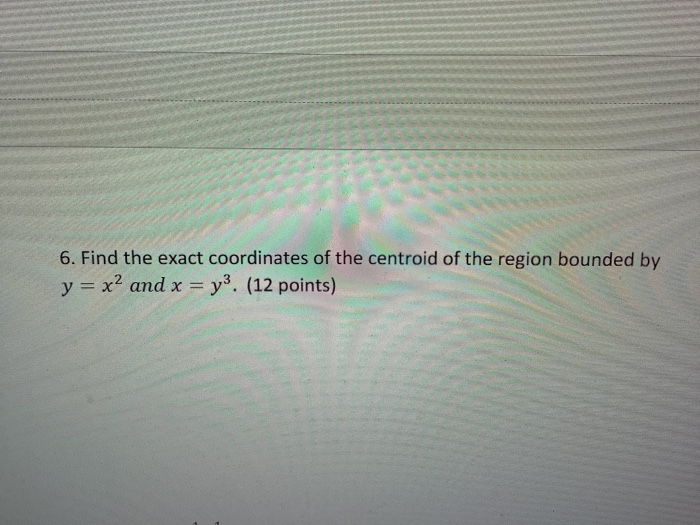6. Find the exact coordinates of the centroid of the region bounded by y = x2 and x = y3. (12 points)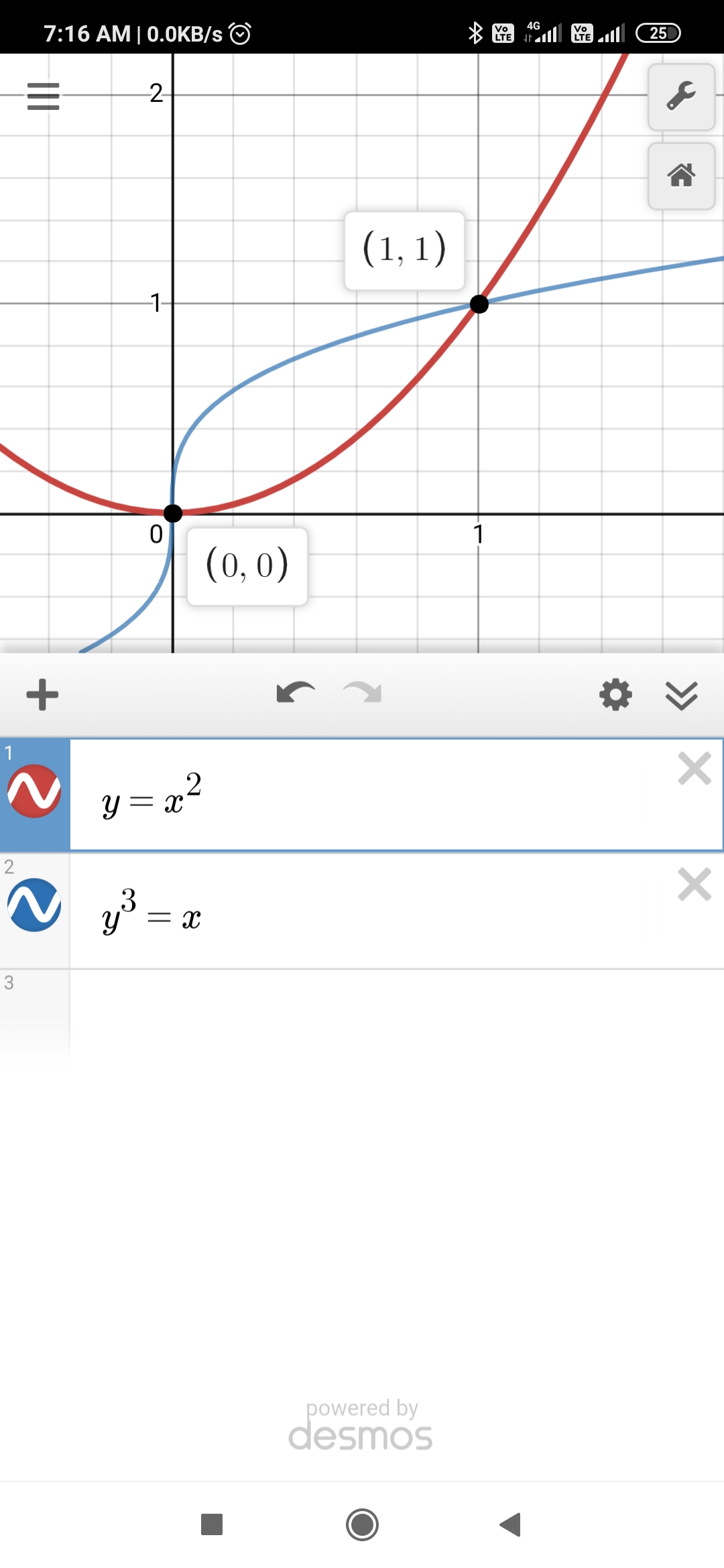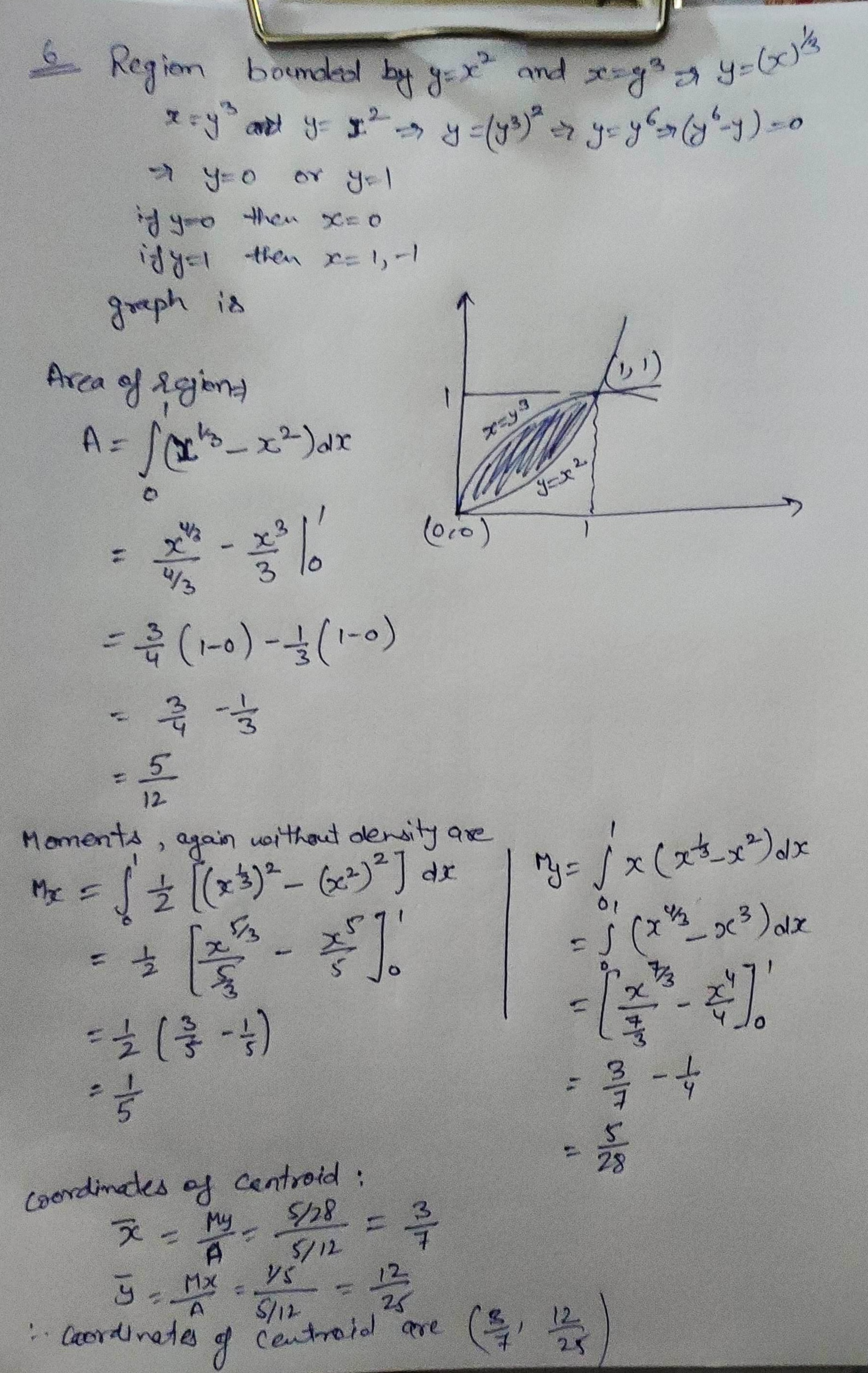##### Add Answer of: 6. Find the exact coordinates of the centroid of the region bounded by y = x2...
Similar Homework Help Questions
• ### 6. Find the exact coordinates of the centroid of the region bounded by y = x?...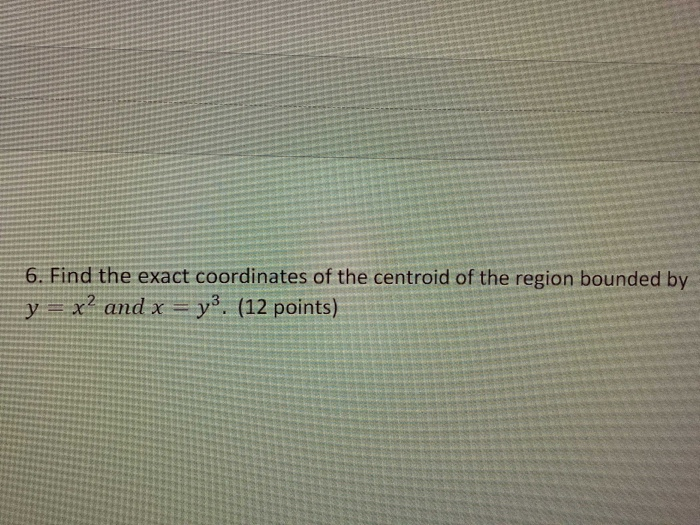6. Find the exact coordinates of the centroid of the region bounded by y = x? and x = y3. (12 points) 1 8. Let f(x) = For what value of c> 0 is fa probability 1+c2x2 density function? (8 points)

• ### Find exact coordinates of the centroid of the region bounded by the given curves.

Find exact coordinates of the centroid of the region bounded by the given curves.y = 3sin(x)y = 3cos(x)x = 0, x = p/4

• ### Find exact coordinates of the centroid of the region bounded by the given curves.

y = 2sin(5x)y = 2cos(5x)x = 0, x = π/20Find exact coordinates of the centroid of the region bounded by the given curves.

• ### Find the x- and y-coordinates of the centroid of the region bounded by the graphs of

Find the x- and y-coordinates of the centroid of the region bounded by the graphs of y=3√x, y=0 and x=8. Sketch the graph.

• ### Find the coordinates of the centroid of the regions bounded by the graphs of: i)y=x2+1,x=0,x=1 and y=0 ii)y+x2=6 and y+2x-3=0

Find the coordinates of the centroid of the regions bounded by the graphs of:i)y=x2+1,x=0,x=1 and y=0ii)y+x2=6 and y+2x-3=0

• ### 6. (20 points) Find the centroid of the region in the first quadrant bounded by the z-axis. 1-y2, and the line x + y 2. 6. (20 points) Find the centroid of the region in the first quadrant bound...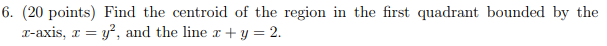6. (20 points) Find the centroid of the region in the first quadrant bounded by the z-axis. 1-y2, and the line x + y 2. 6. (20 points) Find the centroid of the region in the first quadrant bounded by the z-axis. 1-y2, and the line x + y 2.

• ### Find the centroid of the region bounded by y = {x + Ź, y = x”,...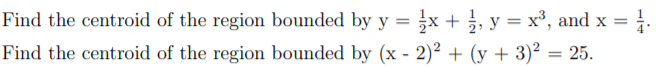Find the centroid of the region bounded by y = {x + Ź, y = x”, and x = 1 Find the centroid of the region bounded by (x - 2)2 + (y + 3)2 = 25.

• ### Use spherical coordinates. Find the centroid of the solid E that is bounded by the xz-plane and t...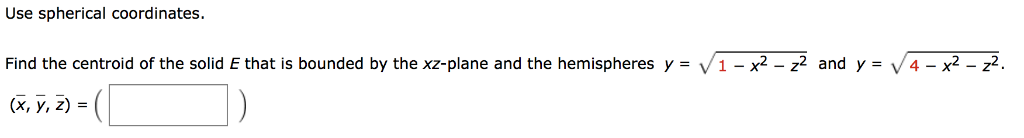Use spherical coordinates. Find the centroid of the solid E that is bounded by the xz-plane and the hemispheres y = V 1-x2-z2 and y (x, y, z) V4-x2- Use spherical coordinates. Find the centroid of the solid E that is bounded by the xz-plane and the hemispheres y = V 1-x2-z2 and y (x, y, z) V4-x2-

• ### The coordinates of the centroid are(x,y)=?

Find the centroid of the region in the first quadrant bounded by the x axis, the parabola y^2=8x, and the line x+y=6The coordinates of the centroid are(x,y)= ?(Simplify your answer. Type an ordered pair. type simplified fractions.)

• ### coordinates of the centroid

Find exact coordinates of the centroid of the region bounded by thegiven curves.y = 3sin(4x)y = 3cos(4x)x = 0, x = π/16im not really sure how to approach this problem

Free Homework App